summary of site-wide JavaScript functionalityUnited States-English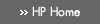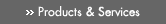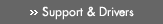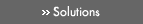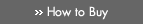» Contact HPSearch:More optionsManualTechnical documentation - EnglishAll of HP USHP-UX Reference > A# atan2d(3M)

HP-UX 11i Version 3: February 2007»

## Technical documentation» Feedback## » Index## NAME

atan2d(), atan2df(), atan2dl(), atan2dw(), atan2dq() — degree-valued arctangent-and-quadrant functions

## SYNOPSIS

#include <math.h>

double atan2d(double y, double x);

float atan2df(float y, float x);

### HP Integrity Server Only

long double atan2dl(long double y, long double x);

extended atan2dw(extended y, extended x);

## DESCRIPTION

atan2d() is a degree-valued version of the atan2() function. It returns the arctangent of y/x, in the range -180 to 180, using the signs of both arguments to determine the quadrant of the return value.

atan2df() is a float version of atan2d(); it takes float arguments and returns a float result.

### Integrity Server Only

atan2dl() is a long double version of atan2d(); it takes long double arguments and returns a long double result.

atan2dw() is an extended version of atan2d(); it takes extended arguments and returns an extended result.

atan2dq() is equivalent to atan2dl() on HP-UX systems.

## USAGE

To use these functions compile either with the default -Ae option or with the -Aa and the -D_HPUX_SOURCE options.

To use (for Integrity servers) atan2dw() or atan2dq(), compile also with the -fpwidetypes option.

To use any of these functions, make sure your program includes <math.h>, and link in the math library by specifying -lm on the compiler or linker command line.

For more information, see the HP-UX floating-point guide for HP Integrity servers at the following site: http://www.hp.com/go/fp.

## RETURN VALUE

If y is ±0 and x is -0, atan2d() returns ±180 respectively.

If y is ±0 and x is +0, atan2d() returns ±0 respectively.

If y is ±0 and x is less than zero, atan2d() returns ±180 respectively.

If y is ±0 and x is greater than zero, atan2d() returns returns ±0 respectively.

If y is less than zero and x is zero, atan2d() returns -90.

If y is greater than zero and x is zero, atan2d() returns 90.

If y is greater than zero and x is -INFINITY, atan2d() returns 180.

If y is less than zero and x is -INFINITY, atan2d() returns -180.

If y is greater than zero and x is INFINITY, atan2d() returns +0.

If y is less than zero and x is INFINITY, atan2d() returns -0.

If y is ±INFINITY and x is finite, atan2d() returns ±90 respectively.

If y is ±INFINITY and x is -INFINITY, atan2d() returns ±135 respectively.

If y is ±INFINITY and x is +INFINITY, atan2d() returns ±45 respectively.

If x or y is NaN, atan2d() returns NaN.

## ERRORS

No errors are defined.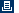Printable versionPrivacy statement Using this site means you accept its terms Feedback to webmaster© 1983-2007 Hewlett-Packard Development Company, L.P.